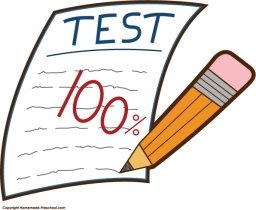# Average score

In a math exam, two students scored 95, six students scored 90, 3 students scored 80, and one students scored 50. What was the average score for the class?Did you find an error or inaccuracy? Feel free to write us. Thank you!Tips to related online calculators
Looking for help with calculating arithmetic mean?
Looking for a statistical calculator?

#### You need to know the following knowledge to solve this word math problem:

We encourage you to watch this tutorial video on this math problem: